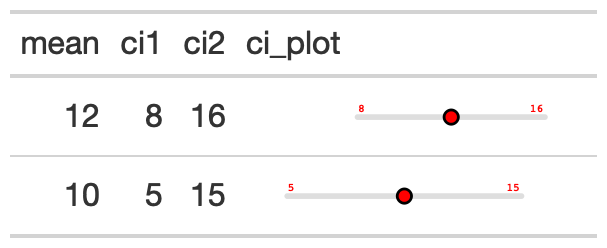Plot a confidence interval around a point

## Usage

gt_plt_conf_int(
gt_object,
column,
ci_columns,
ci = 0.9,
ref_line = NULL,
palette = c("black", "grey", "white", "black"),
width = 45,
text_args = list(accuracy = 1),
text_size = 1.5
)

## Arguments

gt_object

An existing gt table

column

The column that contains the mean of the sample. This can either be a single number per row, if you have calculated the values ahead of time, or a list of values if you want to calculate the confidence intervals.

ci_columns

Optional columns representing the left/right confidence intervals of your sample.

ci

The confidence interval, representing the percentage, ie 0.9 which represents 10-90 for the two tails.

ref_line

A number indicating where to place reference line on x-axis.

palette

A vector of color strings of exactly length 4. The colors represent the central point, the color of the range, the color of the stroke around the central point, and the color of the text, in that specific order.

width

A number indicating the width of the plot in "mm", defaults to 45.

text_args

A list of named arguments. Optional text arguments passed as a list to scales::label_number.

text_size

A number indicating the size of the text indicators in the plot. Defaults to 1.5. Can also be set to 0 to "remove" the text itself.

a gt table

## Examples

# gtExtras can calculate basic conf int
# using confint() function

ci_table <- generate_df(
n = 50, n_grps = 3,
mean = c(10, 15, 20), sd = c(10, 10, 10),
with_seed = 37
) %>%
dplyr::group_by(grp) %>%
dplyr::summarise(
n = dplyr::n(),
avg = mean(values),
sd = sd(values),
list_data = list(values)
) %>%
gt::gt() %>%
gt_plt_conf_int(list_data, ci = 0.9)

# You can also provide your own values
# based on your own algorithm/calculations
pre_calc_ci_tab <- dplyr::tibble(
mean = c(12, 10), ci1 = c(8, 5), ci2 = c(16, 15),
ci_plot = c(12, 10)
) %>%
gt::gt() %>%
gt_plt_conf_int(
ci_plot, c(ci1, ci2),
palette = c("red", "lightgrey", "black", "red")
)

## Figures## Function ID

3-10

Other Themes: gt_plt_bullet(), gt_plt_dot(), gt_theme_538(), gt_theme_dark(), gt_theme_dot_matrix(), gt_theme_espn(), gt_theme_excel(), gt_theme_guardian(), gt_theme_nytimes(), gt_theme_pff()